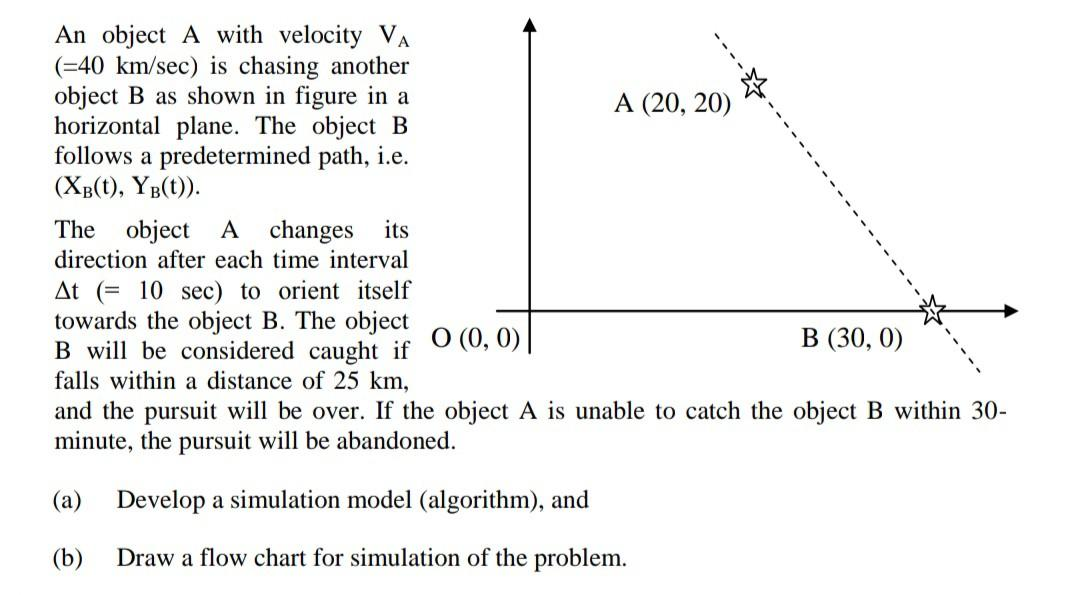# An object A with velocity VA (=40 km/sec) is chasing another object B as shown in figure in a A (20, 20) horizontal plane. The object B follows a predetermined path, i.e. (Xb(t), YB(t)). The object A changes its direction after each time interval At (= 10 sec) to orient itself towards the object B. The object B will be considered caught if O (0,0) | B (30, 0) falls within a distance of 25 km, and the pursuit will be over. If the object A is unable to catch the object B within 30- minute, the pursuit will be abandoned. (a) Develop a simulation model (algorithm), and (b) Draw a flow chart for simulation of the problem.

Question-AnswerCategory: Modelling & SimulationAn object A with velocity VA (=40 km/sec) is chasing another object B as shown in figure in a A (20, 20) horizontal plane. The object B follows a predetermined path, i.e. (Xb(t), YB(t)). The object A changes its direction after each time interval At (= 10 sec) to orient itself towards the object B. The object B will be considered caught if O (0,0) | B (30, 0) falls within a distance of 25 km, and the pursuit will be over. If the object A is unable to catch the object B within 30- minute, the pursuit will be abandoned. (a) Develop a simulation model (algorithm), and (b) Draw a flow chart for simulation of the problem.

An object A with velocity VA (=40 km/sec) is chasing another object B as shown in figure in a A (20, 20) horizontal plane. The object B follows a predetermined path, i.e. (Xb(t), YB(t)). The object A changes its direction after each time interval At (= 10 sec) to orient itself towards the object B. The object B will be considered caught if O (0,0) | B (30, 0) falls within a distance of 25 km, and the pursuit will be over. If the object A is unable to catch the object B within 30- minute, the pursuit will be abandoned.
(a) Develop a simulation model (algorithm), and
(b) Draw a flow chart for simulation of the problem.